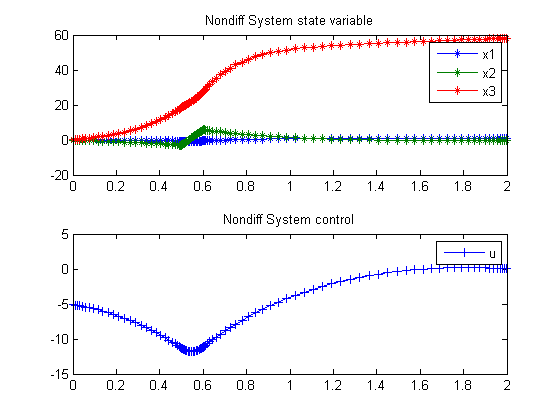72  Nondifferentiable system

Global Optimization of Chemical Processes using Stochastic Algorithms 1996, Julio R. Banga, Warren D. Seider

Case Study IV: Optimal control of a nondifferentiable system

72.1  Problem description

This problem has been studied by Thomopoulos and Papadakis who report convergence difficulties using several optimization algorithms and by Luus using Iterative Dynamic Programming. The optimal control problem is:

Find u(t) to minimize:

 J = x3(tf)

Subject to:

 dx1 dt
= x2
 dx2 dt
= −x1x2+u+d
 dx3 dt
= 5*x12+2.5*x22+0.5*u2

with

 d = 100*[U(t−0.5)−U(t−0.6)]

where U(t-alpha) is the unit function such that U = 0 for t - alpha < 0 and U = 1 for t - alpha > 0. Hence d is a rectangular pulse of magnitude 100 from t=0.5 until t=0.6. These authors consider t_f = 2.0s and the initial conditions:

 x(t0) = [0  0  0]′

Reference: 

72.2  Problem setup

toms t1
p1 = tomPhase('p1', t1, 0, 0.5, 30);
toms t2
p2 = tomPhase('p2', t2, 0.5, 0.1, 20);
toms t3
p3 = tomPhase('p3', t3, 0.6, 2-0.6, 50);

x1p1 = tomState(p1,'x1p1');
x2p1 = tomState(p1,'x2p1');
x3p1 = tomState(p1,'x3p1');
up1 = tomControl(p1,'up1');

x1p2 = tomState(p2,'x1p2');
x2p2 = tomState(p2,'x2p2');
x3p2 = tomState(p2,'x3p2');
up2 = tomControl(p2,'up2');

x1p3 = tomState(p3,'x1p3');
x2p3 = tomState(p3,'x2p3');
x3p3 = tomState(p3,'x3p3');
up3 = tomControl(p3,'up3');

% Initial guess
x0 = {icollocate(p1,{x1p1 == 0;x2p1 == 0;x3p1 == 0})
icollocate(p2,{x1p2 == 0;x2p2 == 0;x3p2 == 0})
icollocate(p3,{x1p3 == 0;x2p3 == 0;x3p3 == 0})
collocate(p1,up1==-4-8*t1/0.5)
collocate(p2,up2==-12)
collocate(p3,up3==-12+14*t3/2)};

% Box constraints
cbox = {
icollocate(p1,{-100 <= x1p1 <= 100
-100 <= x2p1 <= 100
-100 <= x3p1 <= 100})
icollocate(p2,{-100 <= x1p2 <= 100
-100 <= x2p2 <= 100
-100 <= x3p2 <= 100})
icollocate(p3,{-100 <= x1p3 <= 100
-100 <= x2p3 <= 100
-100 <= x3p3 <= 100})
collocate(p1,-15 <= up1 <= 2)
collocate(p2,-15 <= up2 <= 2)
collocate(p3,-15 <= up3 <= 2)};

% Boundary constraints
cbnd = {initial(p1,{x1p1 == 0;x2p1 == 0;x3p1 == 0})
final(p3,x3p3) <= 60};

% ODEs and path constraints
ceq = {collocate(p1,{
dot(p1,x1p1) == x2p1
dot(p1,x2p1) == -x1p1-x2p1+up1
dot(p1,x3p1) == 5*x1p1.^2+2.5*x2p1.^2+0.5*up1.^2})
collocate(p2,{
dot(p2,x1p2) == x2p2
dot(p2,x2p2) == -x1p2-x2p2+up2+100
dot(p2,x3p2) == 5*x1p2.^2+2.5*x2p2.^2+0.5*up2.^2})
collocate(p3,{
dot(p3,x1p3) == x2p3
dot(p3,x2p3) == -x1p3-x2p3+up3
dot(p3,x3p3) == 5*x1p3.^2+2.5*x2p3.^2+0.5*up3.^2})};

% Objective
objective = final(p3,x3p3);

final(p1,x2p1) == initial(p2,x2p2)
final(p1,x3p1) == initial(p2,x3p2)
final(p2,x1p2) == initial(p3,x1p3)
final(p2,x2p2) == initial(p3,x2p3)
final(p2,x3p2) == initial(p3,x3p3)};

72.3  Solve the problem

options = struct;
options.name = 'Nondiff System';
constr = {cbox, cbnd, ceq, link};
solution = ezsolve(objective, constr, x0, options);

t = subs(collocate(p1,t1),solution);
t = [t;subs(collocate(p2,t2),solution)];
t = [t;subs(collocate(p3,t3),solution)];
x1 = subs(collocate(p1,x1p1),solution);
x1 = [x1;subs(collocate(p2,x1p2),solution)];
x1 = [x1;subs(collocate(p3,x1p3),solution)];
x2 = subs(collocate(p1,x2p1),solution);
x2 = [x2;subs(collocate(p2,x2p2),solution)];
x2 = [x2;subs(collocate(p3,x2p3),solution)];
x3 = subs(collocate(p1,x3p1),solution);
x3 = [x3;subs(collocate(p2,x3p2),solution)];
x3 = [x3;subs(collocate(p3,x3p3),solution)];
u = subs(collocate(p1,up1),solution);
u = [u;subs(collocate(p2,up2),solution)];
u = [u;subs(collocate(p3,up3),solution)];
Problem type appears to be: lpcon
Starting numeric solver
===== * * * =================================================================== * * *
TOMLAB - Tomlab Optimization Inc. Development license  999001. Valid to 2011-02-05
=====================================================================================
Problem: ---  1: Nondiff System                 f_k      58.065028469582764000
sum(|constr|)      0.000030760875102477
f(x_k) + sum(|constr|)     58.065059230457869000
f(x_0)      0.000000000000000000

Solver: snopt.  EXIT=0.  INFORM=1.
SNOPT 7.2-5 NLP code
Optimality conditions satisfied

FuncEv    1 ConstrEv   45 ConJacEv   45 Iter   38 MinorIter 1056
CPU time: 1.437500 sec. Elapsed time: 1.516000 sec.

72.4  Plot result

subplot(2,1,1)
plot(t,x1,'*-',t,x2,'*-',t,x3,'*-');
legend('x1','x2','x3');
title('Nondiff System state variable');

subplot(2,1,2)
plot(t,u,'+-');
legend('u');
title('Nondiff System control');